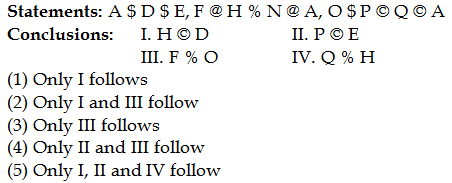## PREPARATORY QUESTIONS

### EQUAL-INEQUAL

#### PREPARATORY SET-33 (HARD)

Direction (Qs.1 to 5): In each of the following questions, a relationship between different elements is shown in the statements. The statements are followed by two conclusions I, II, III & IV. Assuming the given statements to be true, find out which of the conclusions given below is/are definitely true.

‘A \$ B’ means ‘A is not smaller than B’

‘A @ B’ means ‘A is not greater than B’

‘A © B’ means ‘A is neither smaller than nor equal to B’

‘A % B’ means ‘A is neither greater than nor equal to B’

‘A # B’ means ‘A is neither smaller than nor greater than B’

Question No : 1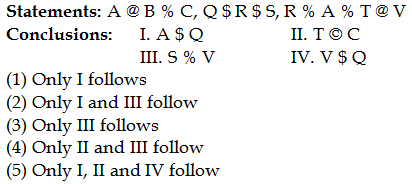Question No : 2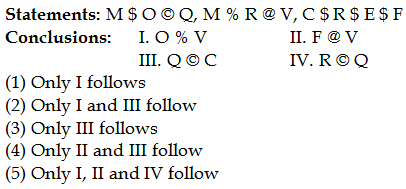Question No : 3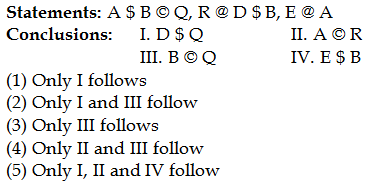Question No : 4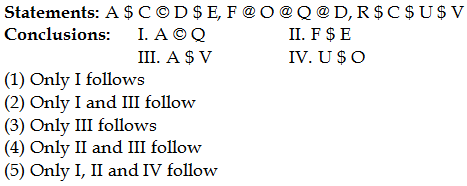Question No : 5